# Effect of temperature on dynamic behavior of cracked metallic and composite beam

## Mainul Hossain1, Jaan Lellep2

1, 2University of Tartu, Tartu, Estonia

1Corresponding author

Vibroengineering PROCEDIA, Vol. 32, 2020, p. 172-178. https://doi.org/10.21595/vp.2020.21337
Received 14 February 2020; received in revised form 3 March 2020; accepted 10 March 2020; published 29 June 2020

46th International Conference on Vibroengineering in St. Petersburg, Russia, June 29 - July 1, 2020

Copyright © 2020 Mainul Hossain, et al. This is an open access article distributed under the Creative Commons Attribution License, which permits unrestricted use, distribution, and reproduction in any medium, provided the original work is properly cited.
Views 90
CrossRef Citations 0
Abstract.

Euler-Bernoulli beam model is used to analyze the thermal vibration of cracked metallic and laminated composite beam with fully clamped ends. An analytical solution technique is proposed to investigate the natural vibration of cracked beam subjected to axial thermal load. An open and stable crack is considered perpendicular to the beam axis. In this study, three different types of material such as steel, copper and aluminum and their composition are used to analyze the thermal effect on different modes of frequency. The effects of thermal stress on the vibration of cracked metallic and composite fully clamped beam are revealed. The results show that the effect of temperature is significant on the natural frequency of cracked metallic and composite beam.

Highlights
• The effect of thermal vibration depends on length to height ratio of the beam
• The effect of temperature is more significant in first mode of frequency
• Under thermal load composite beam is very effective than metallic beam

Keywords: effect of temperature, natural frequency, crack, composite, beam.

#### 1. Introduction

Effect of temperature is one of the important features for designing of structures efficiently. Unfortunately, thermal effect is ignored in most of the research which leads to incorrect results. A small change in temperature may cause a large variation in the behavior of beam vibration . Thermal stresses are considered as mechanical stresses of the elements. It is the result of contraction or expansion of structural elements due to the applied thermal load. When an element is subjected to the rise of temperature it expands and when it loses temperature, it contracts. The expansion and contraction of the element due to the temperature is proportional to the change of temperature. This proportionality depends on the coefficient of linear thermal expansion of the material . According to the theory of linear thermo-elasticity, the thermal strain is linearly added with the mechanical strain. That is why the equations of equilibrium and compatibility of thermal problems are the same as the elasticity problem. According to this assumption, many techniques that have been used to solve the elasticity problems are applicable to the thermo-elasticity problem as well .

Interest in multifunctional composite materials has increased rapidly in recent years. Laminated composite materials are also a very efficient type of composition. Laminated composite materials are formed by different layers of two or more materials that are effectively bonded together. It is a combination of a large number of fibers in a thin layer of matrix. Fibers in the lamina may be continuous or discontinuous, arranged in a specific direction or in a random orientation . Lamination is a very useful technique to achieve more efficient material. Lamination can emphasize the properties of material such as strength, stiffness, low weight, corrosion resistance, thermal insulation, etc. [5-7].

The effect of thermal vibration on the cracked composite beam is very rare in the open literature. Therefore, thermal effects on macro mechanics also achieve substantial attention among researchers. Zhang et al.  studied the thermal effects on high-frequency vibration of beams. Their results showed that the spatial distributions and levels of energy density can be affected by the thermal effects. Cui and Hu  examined thermal buckling and natural vibration of the beam with an axial stick-slip-stop boundary. Lia and Zhang  investigated the thermal effect on vibration and buckling analysis of thin plates with cracks. Their results revealed that the dynamic behavior of structural elements is significantly affected by thermal effects.

In this paper, the effects of temperature on the dynamic behavior of cracked metallic and composite beam are investigated. Our main concern is to compare the effectiveness of a fully clamped metallic and composite beam under crack and temperature. The governing differential equations with boundary and intermediate conditions are solved using analytical techniques. The effects of crack severity, crack position and beam length to height ratio on the frequencies of the heated beam are investigated. On the other hand, the effect of temperature on the vibration of the cracked composite beam is analyzed and compared with the metallic beam in detail.

#### 2. Settings of the problem

A diagram of a cracked beam is illustrated in Fig. 1. The cross section of the beam is rectangular. $L$ is the length and $b$ is the width of the beam respectively. Beam is isotropic and homogeneous where $\rho$ is the density of the metallic beam. Single crack is placed at the location of $x=a$ of the beam. The crack is considered as stable crack whose depth is $c$ and $s$ is the ratio of crack height and beam height. Three different types of material (Aluminium, Copper, and Steel) are considered for the metallic beam as shown in Table 1.

A composite laminated material is also considered for the beam which is formed by three different materials such as aluminum, copper, and steel in a certain combination.

Fig. 1. Geometry of the problem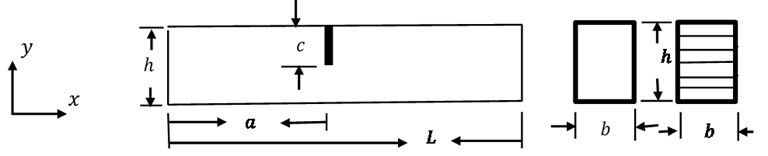Table 1. Material properties

 Material Young’s modulus (GPa) Coefficient of thermal expansion (°C) Density (kg/m3) Aluminium 69 24x10-6 2780 Copper 117 17x10-6 8960 Steel 200 13x10-6 8050

#### 3. Mathematical formulation

A beam is considered along the $X$ axis where transverse deflection $W\left(X,t\right)$ is described along the $Y$ axis. According to the theory of Euler-Bernoulli for small deflection, the equation of motion expressed by the transverse deflection $W\left(X,t\right)$ can be obtained as:

(1)
$EI\frac{{\partial }^{4}W}{\partial {X}^{4}}+\rho A\frac{{\partial }^{2}W}{\partial {t}^{2}}+{N}_{T}\frac{{\partial }^{2}W}{\partial {X}^{2}}=0.$

For separating the variable, this function $W\left(X,t\right)=\stackrel{-}{W}{e}^{i{\omega }_{c}t}$ can be used. Then the Eq. (1) becomes:

(2)
$EI\frac{{\partial }^{4}\stackrel{-}{W}}{\partial {X}^{4}}-\rho A{\omega }_{c}^{2}+{N}_{T}\frac{{\partial }^{2}\stackrel{-}{W}}{\partial {X}^{2}}=0.$

Let us assume that the beam is homogenous having same temperature over its entire length. As a result of thermal expansion, an additional axial force ${N}_{T}$ can be presented as:

(3)
${N}_{T}=-\alpha \theta EA.$

$\alpha$ is the linear thermal extension coefficient, $\theta$ is the temperature difference between the actual and initial temperature. Let us introduce dimensionless variables:

The Eq. (2) becomes:

(4)
$\frac{{\partial }^{4}w}{\partial {x}^{4}}-n\frac{{\partial }^{2}w}{\partial {x}^{2}}-{\omega }^{2}=0.$

Using this function $w\left(x\right)=A{e}^{i\lambda x}$, we can write the characteristic equation as follow:

(5)
${\lambda }^{4}+n{\lambda }^{2}-{\omega }^{2}=0.$

Roots of the equation can be presented as follow:

Solutions of the equation are:

(6)
(7)

Boundary conditions for the fully clamped beam as follow:

(8)

These four boundary conditions and other four intermediate conditions for crack are used to solve the Eqs. (6) and (7). For crack, the stress intensity factor for the single edge notched tension is presented as follow (see ):

(9)

Using the intensity factor intermediate conditions for the crack can be defined as below:

(10)

In composite beam, the Young’s modulus of composite system can be written as:

(11)

Thermal expansion coefficient of composite material can be presented as:

(12)
$\alpha =\frac{{E}_{s}{\alpha }_{s}{V}_{s}+{E}_{a}{\alpha }_{a}{V}_{a}+{E}_{c}{\alpha }_{c}{V}_{c}}{{E}_{s}{V}_{s}+{E}_{a}{V}_{a}+{E}_{c}{V}_{c}}.$

#### 4. Results and discussion

The temperature effect on the vibration of metallic and composite beams is significant. To develop a reliable model to analyse thermal effect on metallic and composite beam are also very essential. In this section, the outcomes of this analysis are presented with graphs and tables. Three different materials such as steel, copper, aluminium and their composite are used in this analysis. In composite, these three materials such as steel, copper, and aluminium are used as a ratio of 40 %, 30 %, and 30 % respectively. The results of this analysis show good accordance with the papers of other researcher in open literature.

Table 2. Natural frequency of solid beam with clamped ends

 1 Frequency in different modes 1 2 3 4 5 6 Current work 22.095 61.295 120.49 199.44 298.09 417.19 Jiang and Wang  21.736 57.967 107.74 166.61 231.10 298.71

Table 2 illustrates the dimensionless natural frequency of fully clamped solid beam. Current research data show good agreement with data of other researcher  in lower mode of frequency. In current work, Euler-Bernoulli theory is used. On the other hand, Jiang and Wang  used nonlocal theory of elasticity with nonlocal parameter value 0.05. That is why, there are some differences in both data sets in higher modes of frequency.

Table 3. Natural frequency of solid beam for different axial loads

 Frequency in different modes $n$ 1 2 3 4 5 6 0 22.37383 61.67188 120.9063 199.8543 298.5484 417.6172 2 21.81445 60.91797 120.0859 198.9844 297.6367 416.7578 4 21.24023 60.16211 119.2523 198.1406 296.7656 415.9063 8 20.04102 58.60547 117.5859 196.3945 294.9844 414.1328 16 17.36621 55.35547 114.1641 192.8613 291.3594 410.6563 32 9.87793 48.15234 106.998 185.6094 284.0156 403.7109

Table 3 depicts the natural frequency of solid beam under dimensionless axial loads. Natural frequency decreases with increase of axial load. Axial load is very effective on the 1st mode of frequency instead of higher mode of frequency.

Table 4. Natural frequency of composite beam for different temperatures

 $L/h$=20 Aluminum (30%), Copper (30%), Steel (40%) Aluminum (40%), Copper (30%), Steel (30%) Aluminum (30%), Copper (40%), Steel (30%) $\theta$ 1 2 3 1 2 3 1 2 3 0 22.3730 61.6738 120.898 22.3744 61.6736 120.898 22.373 61.6738 120.898 5 22.2676 61.5352 120.742 22.2637 61.5273 120.742 22.2676 61.5273 120.742 10 22.1660 61.3867 120.602 22.1543 61.377 120.572 22.1582 61.3789 120.586 20 21.9551 61.1055 120.289 21.929 61.0723 120.246 21.9395 61.0898 120.258 30 21.7402 60.8242 119.977 21.7046 60.7695 119.914 21.7207 60.793 119.945 40 21.5254 60.5352 119.668 21.4746 60.4727 119.586 21.498 60.4961 119.617 50 21.3076 60.2461 119.345 21.2441 60.1602 119.254 21.2715 60.1992 119.305

Table 4 reveals the effect of temperature on natural frequency of composite beam. Three different types of materials such as steel, copper and aluminum are used in these composites. Three different composition ratios of these materials are used to create three different composites. Table data show that increase of temperature decreases frequency. On the other hand, ratio of these compositions is not very significant on natural frequency.

Fig. 2. Frequency versus change of temperature and length to height ratio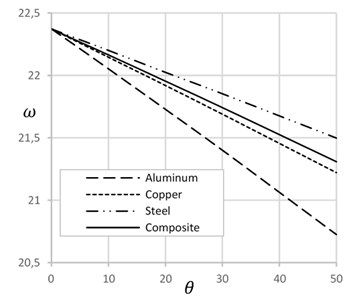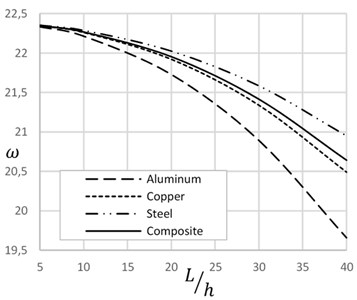Fig. 2 illustrates the effect of temperature and length to height ratio on the natural frequency of beam for different materials. Natural frequency decreases with increase of temperature and the length to height ratio. Temperature increases axial tension that decreases natural frequency. Similarly, increase of length to height ratio decreases height and cross sectional area of beam that increases axial stress. Increase of axial stress decreases natural frequency. Composite material shows the effective results considering the behavior of other materials.

Fig. 3 displays the effect of crack depth ratio on the natural frequency presence and absence of temperature. This figure depicts that increase of crack depth ratio decreases natural frequency. On the absence of temperature, the effect of crack depth ratio is more significant than the presence of that. High temperature expands the length of the beam and reduces the width of the crack that decreases slightly the effect of crack on frequency.

Fig. 4 demonstrates the effect of temperature on the frequency of cracked beams with different crack-depth ratio. At the low crack depth ratio, frequency decreases with the increase of temperature. On the other hand, at the high crack depth ratio, frequency increases with the increase of temperature. Increase of temperature expands the length of the beam and decreases the width of the crack that enhances the frequency of the beam. Fig. 5 describes the effect of crack location on the natural frequency of beam on presence and absence of temperature. Crack position at the ends and at the mid of the beam show lower frequency. On the other hand, in between crack position shows higher frequency of the beam. Frequency of crack beam with temperature is more significant than the crack without temperature.

Fig. 3. Frequency versus crack depth ratio with and without temperature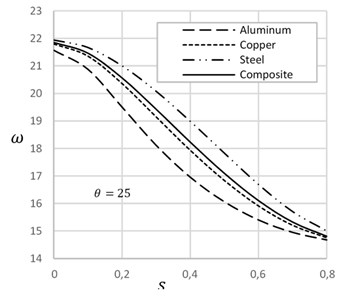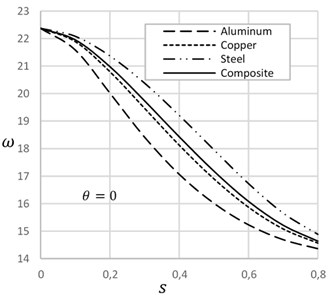Fig. 4. Frequency versus change of temperature for different crack depth ratios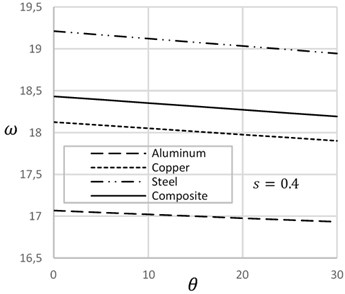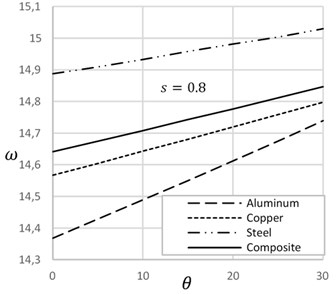Fig. 5. Frequency versus crack location with and without temperature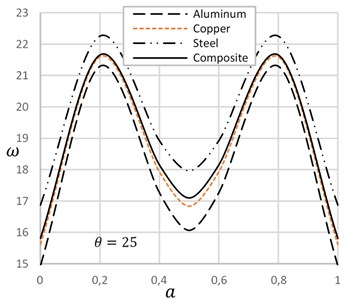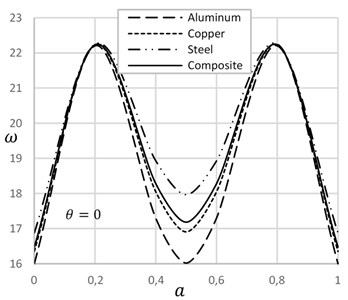#### 5. Conclusions

In this study, Euler-Bernoulli beam theory is employed to develop an analytical technique for analyzing thermal effect on dynamic behavior of cracked metallic and composite beam. The results are presented for three different types of material and their composite. The results of this investigation showed that the effect of thermal vibration depends on length to height ratio of the beam. The effect of temperature is more significant in first mode of frequency. In higher mode of frequency, the temperature effect is less significant. Under thermal load composite beam is very effective than metallic beam.

#### Acknowledgements

The partial support from Estonian Doctoral School of Mathematics and Statistics is acknowledged.

1. Avsec J., Oblak M. Thermal vibrational analysis for simply supported beam and clamped beam. Journal of Sound and Vibration, Vol. 308, 2007, p. 514-525. [Publisher]
2. Barron R. F., Barron B. R. Design for Thermal Stresses. John Wiley and Sons, 2012. [Publisher]
3. Hetnarski R. B., Eslami M. R. Thermal Stresses-Advanced Theory and Applications. Springer Science+Business Media, 2009. [Search CrossRef]
4. Mukhopadhyay M. Composite Materials and Structures. Universities Press (India) Private Limited, 2004. [Search CrossRef]
5. Robert M. J. Mechanics of Composite Materials. Taylor and Francis, 1999. [Search CrossRef]
6. Vasiliev V. V. Advanced Mechanics of Composite Materials and Structural Elements. Elsevier, 2013. [Publisher]
7. Gibson R. F. Principles of Composite Material Mechanics. CRC Press, Taylor and Francis Group, 2012. [Publisher]
8. Zhang W., Chen H., Zhu D., Kong X. The thermal effects on high-frequency vibration of beams using energy flow analysis. Journal of Sound and Vibration, Vol. 333, 2014, p. 2588-2600. [Publisher]
9. Cui D. F., Hu H. Y. Thermal buckling and natural vibration of the beam with an axial stick-slip-stop boundary. Journal of Sound and Vibration, Vol. 333, 2014, p. 2271-2282. [Publisher]
10. Lai S. K., Zhang L. H. Thermal effect on vibration and buckling analysis of thin isotropic/orthotropic rectangular plates with crack defects. Engineering Structures, Vol. 177, 2018, p. 444-458. [Publisher]
11. Anderson T. L. Fracture Mechanics Fundamentals and Applications. CRC Press, Taylor and Francis Group, 2017. [Publisher]
12. Jiang J., Wang L. Analytical solutions for thermal vibration of nanobeams with elastic boundary conditions. Acta Mechanica Solida Sinica, Vol. 30, 2017, p. 474-483. [Publisher]